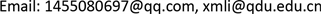﻿ 异方差情形下逆高斯分布的比较 Comparison of several inverse Gaussian populations under heterogeneity

Vol. 08  No. 12 ( 2019 ), Article ID: 33516 , 6 pages
10.12677/AAM.2019.812233

Comparison of Several Inverse Gaussian Populations under Heterogeneity

Ge Qiao, Xinmin Li

Qingdao University, School of Mathematics and Statistics, Qingdao ShandongReceived: 23rd, 2019; accepted: Dec. 11th, 2019; published: Dec. 18th, 2019ABSTRACT

In this paper, we propose a generalized testing procedure for the comparison of multiple inverse Gaussian distributions of heterogeneity, and an extensive numerical simulation is conducted to compare with existing methods under various parameter configurations. The simulation results show that the proposed method has good frequency properties.

Keywords:Inverse Gaussian Distribution, Generalized P Value, Parametric Bootstrap1. 引言

$f\left(x;\mu ,\lambda \right)={\left(\frac{\lambda }{2\pi {x}^{3}}\right)}^{\frac{1}{2}}\mathrm{exp}\left\{\frac{-\lambda {\left(x-\mu \right)}^{2}}{2{\mu }^{2}x}\right\},\text{}x,\mu ,\lambda >\text{0}$

2. 广义检验方法

${\stackrel{¯}{X}}_{i}=\frac{1}{{n}_{i}}\underset{j=1}{\overset{{n}_{i}}{\sum }}{X}_{ij},\text{\hspace{0.17em}}{V}_{i}=\underset{j=1}{\overset{{n}_{i}}{\sum }}\left(\frac{1}{{X}_{ij}}-\frac{1}{{\stackrel{¯}{X}}_{i}}\right),\text{\hspace{0.17em}}1\le i\le k$

${H}_{0}:{\mu }_{1}=\cdots ={\mu }_{k}↔{H}_{1}:{\mu }_{i}\ne {\mu }_{j}\text{forsome}i\ne j$

$H={\left(\begin{array}{ccccc}1& 0& \cdots & 0& -1\\ 0& 1& \cdots & 0& -1\\ ⋮& ⋮& \ddots & ⋮& ⋮\\ 0& 0& \cdots & 1& -1\end{array}\right)}_{\left(k-1\right)×k}$

$\theta =H{\mu }^{-1}$，其中 ${\mu }^{-1}={\left({\mu }_{1}^{-1},\cdots ,{\mu }_{k}^{-1}\right)}^{\prime }$。则上述假设问题可以等价于

${H}_{0}:\theta =0↔{H}_{1}:\theta \ne 0$

${T}_{{\mu }_{i}^{-1}}=\left(\frac{{\stackrel{¯}{X}}_{i}-{\mu }_{i}}{{\mu }_{i}\sqrt{{\stackrel{¯}{X}}_{i}{V}_{i}\text{}/{n}_{i}\left({n}_{i}-1\right)}}\sqrt{\frac{{\stackrel{¯}{x}}_{i}{v}_{i}}{{n}_{i}\left({n}_{i}-1\right)}}+1\right)\cdot \frac{1}{{\stackrel{¯}{x}}_{i}}=\left({W}_{i}\sqrt{\frac{{\stackrel{¯}{x}}_{i}{v}_{i}}{{n}_{i}\left({n}_{i}-1\right)}}+1\right)\cdot \frac{1}{{\stackrel{¯}{x}}_{i}},1\le i\le k$

${T}_{{\mu }_{i}^{-1}}=\left({t}_{{n}_{i}-1}\sqrt{\frac{{\stackrel{¯}{x}}_{i}{v}_{i}}{{n}_{i}\left({n}_{i}-1\right)}}+1\right)\cdot \frac{1}{{\stackrel{¯}{x}}_{i}}$

$E\left({T}_{{\mu }_{i}^{-1}}\right)={x}_{i}^{-1},\text{\hspace{0.17em}}Var\left({T}_{{\mu }_{i}^{-1}}\right)=Var\left({W}_{i}^{2}\right)\cdot \frac{{\stackrel{¯}{x}}_{i}{v}_{i}}{{n}_{i}\left({n}_{i}-1\right)}\cdot \frac{1}{{\stackrel{¯}{x}}_{i}^{2}}=\frac{{v}_{i}}{{n}_{i}\left({n}_{i}-3\right){\stackrel{¯}{x}}_{i}},$

$\begin{array}{c}E\left({T}_{\theta }\right)=E\left(H{T}_{{\mu }^{-1}}\right)=H{\left[E\left({T}_{{\mu }_{1}^{-1}}\right),\cdots ,E\left({T}_{{\mu }_{k}^{-1}}\right)\right]}^{\prime }\\ ={\left({x}_{1}^{-1}-{x}_{k}^{-1},\cdots ,{x}_{k-1}^{-1}-{x}_{k}^{-1}\right)}^{\prime }\end{array}$

$\begin{array}{c}\Sigma =Cov\left({T}_{\theta }\right)=Cov\left(H{T}_{{\mu }^{-1}}\right)=H\cdot Cov\left({T}_{{\mu }^{-1}}\right)\cdot {H}^{\prime }\\ =H\cdot diag\left[Var\left({T}_{{\mu }_{1}^{-1}}\right),\cdots ,Var\left({T}_{{\mu }_{k}^{-1}}\right)\right]\cdot {H}^{\prime }\end{array}$

$p={P}_{r}\left(T\ge t|{H}_{0}\right)={P}_{r}\left(T\ge {t}_{0}\right)$

1) 分别计算 ${\stackrel{¯}{x}}_{i}$${v}_{i}$$1\le i\le k$，得到 $E\left({T}_{\theta }\right)$$\Sigma$${t}_{0}$

2) 产生 ${t}_{i}~t\left({n}_{i}-1\right)$，并且计算 ${T}_{\theta }$ 和T的值。

3) 将第2步重复M次，得到M个T值。

4) 计算T大于等于 ${t}_{0}$ 的比例。

3. 数值模拟

1) 只有当k足够小时，如k = 3的情况下，Tian的检验方法才有效。随着k的增加，其第一类错误概率明显增大，即使提供大样本也无效。

2) 一般来说，PB方法在k值适中的情况下具有令人满意的性能。然而，当k太小或太大时，第一类错误概率会稍微激进一些，比如k = 3或者k = 20。特别是当样本量小的情况下，k值大或小其第一类错误概率都会明显超过名义水平。

3) n的值倾向于影响所提出的广义检验方法的第一类错误概率，而不是k的值。模拟结果表明，当n较小时，新方法的计算结果相对保守。相反，当样本量过大时，第一类错误概率会略高于名义水平。

Table 2. The simulation results about k = 6

na: n = (5,4s,5); nb: n = (5,10,15,5,10,15); nc: n = (10,30,50,10,30,50).

Table3. The simulation results about k = 20

na: n = (5,20 s); nb: n = (5,10,15,20,30, 5,10,15,20,30, 5,10,15,20,30, 5,10,15,20,30); nc: n = (10,20,30,50,80, 10,20,30,50,80, 10,20,30,50,80, 10,20,30,50,80).

4. 结论

IG分布被广泛用于描述和分析正向右偏的数据。在本文中，我们提出了一个广义检验方法。仿真研究表明，当样本量较小时，我们的方法相对保守。但是，当样本量较大时，所给检验方法仍然能够控制第一类错误概率。

Comparison of several inverse Gaussian populations under heterogeneity[J]. 应用数学进展, 2019, 08(12): 2029-2034. https://doi.org/10.12677/AAM.2019.812233

1. 1. Chhikara, R.S. and Folks, J.L. (1989) The Inverse Gaussian Distribution. Marcel Dekker, New York.

2. 2. Seshadri, V. (1993) The Inverse Gaussian Distribution: A Case Study in Exponential Families. Clarendon Press, Oxford.

3. 3. Seshadri V. (1999) The Inverse Gaussian Distribution: Statistical Theory and Applications. Springer, New York.

4. 4. Tian, L. (2006) Testing Equality of Inverse Gaussian Means under Heterogeneity, Based on Generalized Test Variable. Computational Statistics & Data Analysis, 51, 1156-1162.
https://doi.org/10.1016/j.csda.2005.11.012

5. 5. Ma, C. and Tian, L. (2009) A Parametric Bootstrap Approach for Testing Equality of Inverse Gaussian Means under Heterogeneity. Communications in Statistics—Simulation and Computation, 38, 1153-1160.
https://doi.org/10.1080/03610910902833470

6. 6. Chang, M., You, X. and Wen, M. (2012) Testing the Homogeneity of Inverse Gaussian Scale-Like Parameters. Statistics & Probability Letters, 82, 1755-1760.
https://doi.org/10.1016/j.spl.2012.05.013

7. 7. Sadooghi-Alvandi, S.M. and Malekzadeh, A. (2013) A Note on Testing Homogeneity of the Scale Parameters of Several Inverse Gaussian Distributions. Statistics & Probability Letters, 83, 1844-1848.
https://doi.org/10.1016/j.spl.2013.04.019

8. 8. Zhang, G. (2014) Simultaneous Confidence Intervals for Several Inverse Gaussian Populations. Statistics & Probability Letters, 92, 125-131.
https://doi.org/10.1016/j.spl.2014.05.013

9. 9. Weerahandi, S. (1993) Generalized Confidence Intervals. Journal of the American Statistical Association, 88, 899-905.
https://doi.org/10.1080/01621459.1993.10476355

10. 10. Tusi, K.W. and Weerahandi, S. (1989) Generalized p-Values in Significance Testing of Hypotheses in the Presence of Nuisance Parameters. Journal of the American Statistical Association, 84, 602-607.
https://doi.org/10.1080/01621459.1989.10478810